Modeling Flow and Solute Transport in Irrigation Furrows | OMICS International
Irrigation & Drainage Systems Engineering

# Modeling Flow and Solute Transport in Irrigation Furrows

Zerihun D1, Sanchez CA2*, Lazarovitch N3, Warrick AW4, Clemmens AJ5 and Bautista E6

1Maricopa Agricultural Center, University of Arizona, Maricopa, USA

2Department of Soil, Water, and Environmental Sciences and Maricopa Agricultural Center, University of Arizona, Maricopa, Arizona

3Department of Dryland Agriculture, French Associates Institute for Agriculture and Biotechnology of Drylands, Jacob Blaustein Institutes for Desert Research, Ben-Gurion University of the Negev, Sede Boqer Campus, Israel

4Department of Soil, Water, and Environmental Science, University of Arizona, Shantz Bldg, Tucson, USA

5Formerly, USDA-ARS U.S. Arid Lands Agricultural Research Center, 21881 N. Cardon Lane, Maricopa, AZ 85138, USA

6USDA-ARS U.S. Arid Lands Agricultural Research Center, 21881 N. Cardon Lane, Maricopa, AZ 85138, USA

*Corresponding Author:
Charles A Sanchez
Department of Soil, Water
and Environmental Sciences and Maricopa Agricultural Center
University of Arizona, 37860
W. Smith-Enke RD, Maricopa, Arizona
Tel: + 520-568 2273
E-mail: [email protected]

Received June 17, 2014; Accepted June 20, 2014; Published June 28, 2014

Citation: Zerihun D, Sanchez CA, Lazarovitch N, Warrick AW, Clemmens AJ, et al. (2014) Modeling Flow and Solute Transport in Irrigation Furrows. Irrigat Drainage Sys Eng 3:124. doi:10.4172/2168-9768.1000124

Copyright: © 2014 Zerihun D, et al. This is an open-access article distributed under the terms of the Creative Commons Attribution License, which permits unrestricted use, distribution, and reproduction in any medium, provided the original author and source are credited.

Visit for more related articles at Irrigation & Drainage Systems Engineering

#### Abstract

This paper presents an internally coupled flow and solute transport model for free-draining irrigation furrows. Furrow hydraulics is simulated with a numerical zero-inertia model and solute transport is computed with a numerical cross-section averaged advection-dispersion model. A procedure for integrating the furrow volumetric cumulative intake integral in the context of a hydraulic model is presented. Two hydraulic and solute transport data sets collected in sloping free-draining test furrows were used in model evaluation. Soil intake and hydraulic parameters were estimated with a simple approach that matches simulated and measured flow depth hydrographs. The field-scale Weighted Mean Relative Residual (WMRR) between measured and model predicted flow depth hydrographs are 22.0% and 29.0% for the two data set. Furthermore, it is shown that the WMRR of 29.0% reduces to 16.0%, when only the error associated with the downstream end computational node is excluded. This suggests that a significant fraction of the error is related to the form of the downstream boundary condition used. It also shows that the effect of the downstream boundary condition does not extend to a large segment of the flow upstream. The longitudinal dispersion coefficient is approximated with an explicit equation as a function of the hydraulic and geometric variables. Model evaluation is conducted in three steps: (1) cumulative intakes and intake rates computed with the numerical formulation presented here were compared with a subsurface flow model, HYDRUS-2D; (2) solute breakthrough curves computed with the coupled flow and transport model were compared with those from exact analytical solutions for applicable conditions; and (3) model predicted solute breakthrough curves were compared with those obtained from field measurements. Overall the results suggest that the coupled flow and transport model is a useful irrigation and fertigation system management and evaluation tool.

#### Keywords

Furrow; Infiltration modeling; Hydraulic model; Solute transport model; Coupled model

#### Notation

A=cross-sectional area of flow (L2);

Az=volumetric cumulative infiltration per unit length (L3/L);

C=solute concentration (M/L3);

C0=constant solute concentration at furrow inlet (M/L3);

Ci=initial solute concentration in the irrigation stream (M/L3);

K(h)=unsaturated hydraulic conductivity function (L/T);

KS=saturated hydraulic conductivity (L/T);

Kx=hydrodynamic (longitudinal) dispersion coefficient (L2/T);

L=field length (L);

Q=flow rate (L3/T);

Se=effective saturation (-);

So=furrow longitudinal slope (-);

Z=cumulative infiltration depth (L);

b=width of flow (L);

cu=dimensional constant for the Manning formula (L1/2/T);

fc=a constant of the longitudinal dispersion function (-) ;

k(L/Ta), a(-), and b(L/T)=parameters of the modified Kostiakov function;

n=Manning roughness coefficient (L1/6);

t=time referenced from the beginning of irrigation (T);

t1=the preceding time level (t1=tc-Δtc), referenced from advance time to computational node j (T);

tc=current time, referenced from advance time to computational node j (T);

ts1=time referenced from tso (T);

ts0=time at which solute application begins, referenced from the beginning of irrigation (T);

ts2=time referenced from tsco (T);

tsco=time at which solute application ends, referenced from the beginning of irrigation (T);

v=average cross-sectional velocity (L/T);

v*=shear velocity (L/T);

x=distance along the furrow (L);

y=flow depth (L);

Δtc=current time step size, Δtc=tc -t1 (T);

α (1/L), η (-), and m (-)=parameters of the van Genuchten soil water characteristics function;

δpi=length of the ith incremental wetted perimeter (L);

γ1 (L(1-2γ2)), γ2(-), ρ1(L(16-6ρ2)/3)), and ρ2(-)=furrow geometry parameters;

τ1=intake opportunity time at t1 and on the jth node along the furrow (T);

τc=intake opportunity time at tc and on the jth node along the furrow (T);

θ =volumetric soil water content (-);

θi=inital soil water content (-);

θr=residual soil water content (-);

θs=saturation soil water content (-);

#### Introduction

Modeling the transport of solutes in irrigation furrows requires some level of coupling between the solutions of the surface and subsurface flow and transport equations. Numerical solutions of the one-dimensional (1D) open-channel flow equations [1-3] provide the hydrodynamic basis for surface irrigation solute transport models [4-6]. Field-scale physics based surface-subsurface flow and transport modeling of surface irrigation processes is feasible . However, such models require the integration of four sub-modules (surface irrigation hydraulic and transport models and subsurface flow and transport models) interacting at the level of the duration of a time step. Hence, they are computationally intensive.

In surface irrigation modeling, infiltration is typically computed with empirical functions, often using the Kostiakov  and the modified Kostiakov  equation. In irrigation furrows the extent of the infiltrating surface (wetted perimeter) varies both with time and distance. The variation in wetted perimeter has a direct effect on infiltration from a furrow cross-section as well as on the longitudinal distribution of infiltrated water along a furrow [10-12]. In this study, a numerical procedure is presented for integrating a volumetric cumulative intake integral (that takes into account wetted perimeter effects) in the context of a furrow hydraulic model. With this approach the dynamics of subsurface flow and transport is neglected or replaced with simplifying assumptions relating to the distribution of water and solute in the soil profile, but the resulting model is a lot more efficient and robust and is useful in practical irrigation system management.

The cross-section averaged advection-dispersion equation (ADE) is the governing equation for the transport of a nonreactive, nonsorbing, and neutrally buoyant solute in a furrow irrigation stream. The cross-section averaged ADE can be discretized with Eulerian integration schemes . However, such schemes often lead to serious numerical problems in advection dominated transport problems with steep concentration gradients, such as those encountered in furrow irrigation streams. A numerical procedure, known as the split-operator method, which decouples (in the mathematical sense) the advective transport from the mechanism of dispersion and solve the resulting pair of equations in two steps has been shown to be accurate and efficient in modeling advection dominated solute transport processes in free surface flows [13-15], including surface irrigation streams [4,6,7]. The solute transport model described in this paper is an adaptation, for irrigation furrows, of the basin and border model presented in reference .

Results of recent studies  suggest that limitations of the Eulerian methods could be overcome through the discretization of the coupled flow and transport equations with high resolution Total Variation Diminishing (TVD) schemes. Considering that established surface irrigation hydraulic models [1,17] are based on Eulerian numerical schemes (effective for hydraulic modeling but not suitable for solving advection dominated transport problems) there is little prospect that the fully coupled approach will be integrated into existing surface irrigation hydraulic models in the near future. The preceding discussion suggests that empirical infiltration function based internally coupled hydraulic and solute transport models will remain the most promising agricultural systems management and environmental risk assessment tools for some time to come.

This paper presents an internally coupled flow and solute transport model for free-draining irrigation furrows, with sub-modules interacting at the level of the duration of a time step, which represents an advance over the non-coupled furrow irrigation transport models [5,6]. Furrow hydraulics is simulated with a numerical zero-inertia model, adapted from the model described in reference . A numerical procedure is presented for integrating the furrow volumetric cumulative intake integral in the context of a hydraulic model. The solution of the 1D advection-dispersion equation described in reference  is adapted for furrows in the current study. The numerical technique used to solve the flow equations in the coupled model described here is an industry standard for surface irrigation hydraulic modeling systems. Hence, the solution of the solute transport equation can readily be integrated into existing surface irrigation hydraulic models. Model evaluation is conducted in three steps: (1) a numerical approach presented here for computing infiltration from a furrow is evaluated through comparison with HYDRUS-2D ), (2) the numerical solute transport model described here is tested by comparing its output with simplified analytical solutions, both for pure advection and advection-dispersion in a quasi-uniform flow field, and (3) the coupled model is evaluated by comparing field measured and model predicted solute breakthrough curves at a series of points along two test furrows. Hydraulic and solute transport data sets collected in two test furrows at the Desert Research and Extension Centre of the University of California-Davis (labeled as DREC-1 and DREC-2 data sets) were used in model evaluation. Overall the results of model evaluation show that the coupled flow and transport model is a useful predictive tool for irrigation and fertigation system management.

#### Furrow Hydraulic Model

Using the Manning equation to evaluate the friction slope and empirical approximations for flow depth and the hydraulic section factor , the zero-inertia equations for a furrow can be given as: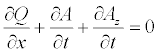(1)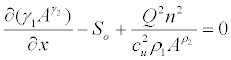(2)

where Q=furrow flow rate (L3/T), x=distance along furrow (L), A=flow cross-sectional area (L2), AZ=volumetric cumulative intake per unit length of furrow (L3/L), t=time (T), γ1 (L(1-2γ2)), γ2 (-), ρ1( L(16-6ρ2)/3)) and ρ2 (-)=empirical furrow geometry parameters, So= furrow longitudinal slope (-), n=Manning roughness coefficient (L1/6), and cu=dimensional constant equivalent to 1 m1/2/s in an appropriate unit (L1/2/T).

Definition of the furrow irrigation hydraulic problem is completed with a statement of pertinent initial and boundary conditions . Much of the boundary and initial conditions are physically intuitive and mathematically trivial. However, the downstream boundary condition for a free-draining furrow during the post-advance phase requires further discussion. A widely used downstream boundary condition for a free-draining furrow is a rating curve (given as QL=f(AL), where AL and QL=cross-sectional area of flow and flow rate, respectively, at the downstream boundary of the computational domain, with the subscript L being the length of the furrow). There are three alternative implementations of such a boundary condition in the model described here: (1) For furrows with a free over-fall downstream boundary, the approach described by Strelkoff  for a free-draining border strip is adapted here for a free-draining furrow. For furrows that do not drain freely into a drainage channel, depending on the availability of data, the rating curve at the downstream boundary of the computational domain can be specified either: (1) based on field measured depth-flow rate data or (2) based on a normal depth assumption. These boundary conditions are useful when there is interest in modeling only a section of the stream, between the inlet end and some point in the middle of the stream, or if the stream does not end in a free over-fall, but instead continue to flow to an adjacent field downstream. Numerical implementation of these boundary conditions, in each iteration of a time step, involves linearization of the Q(AL) function with a first-order approximation of Q, the resulting equation is then integrated into the double-sweep algorithm. Details of the numerical solution of Equations 1 and 2 are given in references [3,20,21].

Furrow infiltration modeling

Unlike basins and borders; furrows have much narrower crosssection, with flow depth and width that are of the same order of magnitude. Hence, flow depth variation in a furrow not only affects the surface boundary condition for infiltration, but also the extent of the soil-water interface (wetted perimeter) through which infiltration occurs. Considering a homogeneous, isotropic soil, and a uniform initial water content; at any one time in the course of an irrigation event the soil water content distribution, (hence matric head gradient) about a point along a furrow wetted perimeter is nonsymmetrical. The nonsymmetrical distribution of soil water about a point along a furrow cross-section produces the two-dimensional infiltration flux, i2D, at the point. Physics based reasoning suggests that the angle that i2D makes with the vertical, measured in the counter-clockwise direction, changes continuously with intake opportunity time at the point (as the soil water content increases). It is maximum when a point is first wetted (and could be well above 90°) and steadily decreases to a minimum of about 0° (oriented vertically downward) after a sufficiently large time following initial wetting. The preceding discussion shows that both the magnitude and the direction of i2D vary along the wetted perimeter of the furrow.

Given a computational node along a furrow (say node j), at any given time, t (referenced from the advance time to node j, taj), the volumetric infiltration rate can be expressed as the line integral of the two-dimensional infiltration flux, i2D (L/T), along the wetted perimeter Figures 1a-1d: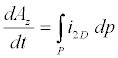(3)

Where dp=differential arc length along the wetted perimeter (L) and P=the wetted perimeter curve. Equation 3 is the statement of continuity for furrow infiltration in its fundamental form - the flux integral is equated with change in storage. At this level of approximation, the 2D infiltration flux, i2D, can in theory be derived from a rigorous physics based model or some approximations thereof. In which case at any given point on the wetted perimeter, i2D is some function of the soil hydraulic properties and initial and boundary conditions. However, empirical approximations can be used, in which case given a soil type and initial and boundary conditions (the effect of which are taken into account by model parameters in an average sense), i2D depends only on the intake opportunity time, τp, which is variable along the wetted perimeter. Based on empirical approximation of i2D, it can be shown that an integral expression for the volumetric cumulative intake from a furrow cross-section (a form amenable to numerical solution) can be derived from the continuity equation.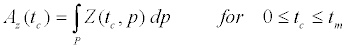(4)

Where tc=current time referenced from advance time to a computational node Figure 1a-1d; Z=cumulative infiltration depth at a point, p, along the wetted perimeter in a furrow cross-section (L); p=is a point on the wetted perimeter, defined in terms of its horizontal and vertical coordinates; and tm=time at which flow depth at a furrow crosssection is maximum, referenced from advance time to a computational node.

Figure 1: (a) Discretization of the solution domain for a numerical solution of Equations 1 and 2, (b) Flow depth hydrograph at computational node j, (c) Furrow wettedperimeter as a function of time at node j, and (d) Cumulative intake as a function of time at node j (t=time measured from the beginning of irrigation, x=distance alongfurrow measured from inlet end, i=index of nodal wetted perimeter increments, I=total number of wetted perimeter increments at the current time, tc, tc=current timereferenced from taj, taj=advance time to node j, j=distance step index, k=time step index, tL=advance time to downstream end, tdp=depletion time, tr0 =recession timeat furrow inlet, y= flow depth hydrograph, ymax=nodal maximum flow depth, p=wetted perimeter as a function of time, pmax=nodal maximum wetted perimeter, and Az=volumetric cumulative intake function).

In Equation 4 the two dimensional nature of infiltration is not explicit, however, it is taken into account (in an average sense) by the infiltration parameter estimates. Noting that Z(tc,p) in Equation 4 is equivalent to Z(τp), whereτp is the intake opportunity time at any given point p along the wetted perimeter at the current time; it follows that Equation 4 is essentially the same as the equation postulated in reference  to define volumetric cumulative intake from a furrow crosssection. As indicated above, Equation 4 is valid only for the advance and wetting phases in a furrow cross-section. It can be shown that the integral expression for the infiltration phase during which water level is decreasing is too complex to be reduced to a simpler form as Equation 4 and it is of no practical computational value. Hence, in the model described here a simpler numerical approximation is used to compute cumulative infiltration during the recession phase. Since the recession phase in irrigation furrows typically accounts for a small fraction of the total irrigation time, this simplification is not a significant limitation.

Numerical integration of the volumetric intake function in the context of a surface irrigation hydraulic model

Some authors [11,12] have examined the relative merits and limitations, in terms of accuracy and robustness, of various numerical approximations to the integral expression in Equation 4 and some additional formulations. These studies noted that some approximations to the integral expression in Equation 4 are computationally intensive and their accuracy is impaired due to loss of significant digits. However, recent advances in computer software and hardware make this issue less important. Hence, more rigorous formulations are explored subsequently.

Assuming the time step sizes, Δt, used in the numerical solution of Equations 1 and 2, Figure 1a-1d are sufficiently small, at any given computational node along the furrow (say node j) and time, tc, the cumulative infiltration depth function Z(tc,p) can be integrated numerically over the wetted perimeter, leading to the form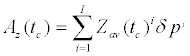(5)

where i=index of nodal wetted perimeter increments at a furrow cross-section (i=1 at the end of the first time step following the arrival of the advancing front at the furrow bottom at node j and is incremented by one at the end of each subsequent time step), I=the total number of wetted perimeter increments on node j at the current time, tc Figure 1b and 1d; Zav(tc)i= the arithmetic average of the cumulative infiltration depths at the upper and lower ends of the ith wetted perimeter increment at time tc (L); and δpi=the ith wetted perimeter increment (L). The cumulative infiltration depth at the ith computational point along the wetted perimeter and at time tc, Z(tc)i, can be calculated with an appropriate cumulative intake function. In the model described here the modified Kostiakov function is used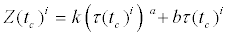(6)

In Equation 6, k (L/Ta), a (-), and b (L/T)=infiltration parameters and τ(tc)i=the intake opportunity time at the ith computational point on the wetted perimeter and time tc. Note that in Equation 6, i is an index for the computational points along the wetted perimeter, in which case i=0 at the furrow bottom and is incremented by one at the upper end of each wetted perimeter increment. Considering a numerical solution of Equations 1 and 2, in which the time step size (Δt) is known (which is the case in the model described here), the cumulative infiltration depth at each of the computational points along the furrow cross-section, Z(tc)i ; the corresponding Zav(tc)i for all the wetted perimeter increments; and all the wetted perimeter increments, δpi, except the Ith increment (i.e., the increment for the current time step) are known. The Ith wetted perimeter increment, δpI, is to be determined as part of the numerical solution of Equations 1 and 2. Hence, Equation 5 can be expressed in terms the incremental wetted perimeter during the current time step as follows: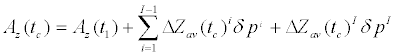(7)

In Equation 7, t1=the preceding time level referenced from advance time to the computational node (tc-Δtc); Δtc=current time step (T); and ΔZav(tc)i=the arithmetic average of the incremental cumulative infiltration depths at the upper and lower ends of the ith wetted perimeter increment during Δtc (L). The first term in Equation 7 represents the volumetric cumulative intake at t1, the second term represents the incremental volumetric cumulative infiltration during Δtc over the wetted perimeter extant at t1, and the third term represent the contribution of the Ith wetted perimeter increment, δpI.

Based on the definition of wetted perimeter, p, and an empirical approximation of the square of the hydraulic section factor, A2R4/3=ρ1 Aρ2; at any given node along the furrow the ith incremental arc length, δpi, can be given in terms of nodal flow cross-sectional area, A: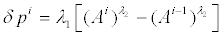(8)

In Equation 8, λ1 (L(6ρ2-16)/4))=(ρ1)-3/4 and λ2 (-)=2.5-0.75ρ2 .

Substituting Equation 8 in 7 and rearranging terms yields the equation for volumetric cumulative infiltration per unit length of furrow as implemented in the hydraulic model described here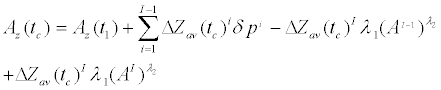(9)

In Equation 9, the first term on the right hand side is the volumetric cumulative intake at t1, the summation (the second) term represents the incremental volumetric cumulative infiltration during the current time step (Δtc) over the wetted perimeter extant at t1, and the sum of the third and fourth terms represent the contribution of the Ith wetted perimeter increment, δpI.

As shown in Equation 4 and 9 is applicable as long as the wetted perimeter is increasing. Attempts to compute the volumetric cumulative infiltration during the period water level is decreasing with a slightly modified version of Equation 9 were not successful due to numerical problems. Hence, in this analysis volumetric cumulative infiltration during the period water level is decreasing is computed with a simpler equation .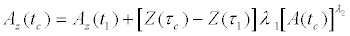(10)

Where τc=tc-taj, τ1=t1-taj, and taj=advance time to node j (T). The first term on the right hand side of Equation 10 represents volumetric cumulative intake at t1 and the second term represents the incremental nodal volumetric cumulative intake corresponding to the current time step, Δtc. With Equation10, the incremental volumetric cumulative infiltration depth, over Δtc, is computed as a function of the intake opportunity time at the furrow bottom and the current wetted perimeter. It is important to note that the use of Equation 9 in conjunction with Equation 10 assumes that advance and recession do not occur simultaneously. However, inflow cutoff time in furrows is typically larger than advance time; hence this is not a serious limitation.

#### Solute Transport Equations

The motion of a non-reactive, non-sorbing, and neutrally buoyant solute in a furrow irrigation stream can be described by a first order partial differential equation of the form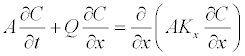(11)

Obtained by combining the conservative form of the cross-section averaged advection-dispersion equation with the continuity equation, Equation 1, of the hydraulic model . In Equation 11, C=solute concentration (M/L3) and Kx=longitudinal dispersion coefficient (L2/T). Flow cross-sectional area, A, and flow rate, Q, are obtained from the solution of the water flow model (Equations 1 and 2). The limiting assumptions that apply to Equation 11 are described in reference . The most significant assumption in the derivation of the crosssection averaged advection-dispersion equation is the description of dispersion as a gradient-diffusion process (that Fick’s law can be used to model hydrodynamic dispersion). This assumption is valid only in the channel segment where differential advection is in equilibrium with turbulent diffusion. In general, the length of the initial advection dominated period (or channel reach), following solute injection, is directly proportional to the square of the channel width . Given that furrows are miniature channels with longitudinal dimension orders of magnitude greater than its vertical and transverse dimensions (and that the transverse and vertical dimensions are of the same order of magnitude), complete mixing in a cross-section can be expected to occur shortly after the injection of solutes at the furrow inlet – at a short distance relative the furrow length. This implies that in theory Equation 11 is well suited to simulating solute transport in irrigation furrows.

Numerical solution of the solute transport equation

In surface irrigation context, where advection is the dominant transport mechanism, an accurate and efficient numerical solution of Equation 11 can be achieved by decoupling the advective transport from the mechanism of dispersion and solving the resulting pair of equations in two steps with numerical techniques appropriate to each sub-problem. Accordingly, Equation 11 can be expressed as a combination of two sub-systems: pure advection (Kx=0) described by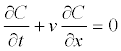(12)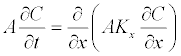(13)

First Equation 12 is solved, for the intermediate solute concentrations, Ca, typically with a semi-Lagrangian integration scheme Holly and Preissmann . The advected concentrations, Ca, are then diffused longitudinally by solving Equation 13 with Eulerian integration schemes [4,6,7]. Pertinent initial and boundary conditions are described in reference .

In the model described here, the semi-Lagrangian integration scheme used to solve Equation 12 implements a combination of the method of characteristics and an interpolation scheme based on Hermite cubic polynomial. For the diffusion step, Equation 13 is recast as a pair of first order linear differential equations. These equations are then discretized using the Preissmann implicit finite difference scheme. The resulting linear equations are then coupled leading to a system of linear algebraic equations. The linear system of equation is solved using the double-sweep algorithm, after being augmented with pertinent initial and boundary conditions.

The Preissmann finite difference approximation is not only robust and accurate (if the solution domain is discretized with prudence), but also handles boundary conditions more effectively than other implicit methods that use three nodes to discretize the spatial derivative. A detailed description of the split-operator method as applied to the solution of Equations 12 and 13 in the model described here can be obtained from reference . In addition to differences in terms of the specific numerical techniques used to solve the advection-dispersion equation, an important advance that distinguishes the model described here from other published furrow irrigation solute transport models [5,6] is that the model presented here is an internally coupled model. Hence, the flow and solute transport components of the model described in this paper are programmatically linked and are interacting at the level of the duration of a time step. This aspect of the flow and transport model is described subsequently.

#### Coupling of the Water Flow and Solute Transport Components of the Model

A description of the approach used for coupling of the hydraulic and solute transport components was provided in reference . A concise review this procedure is presented here.

From programming point of view, the solute transport module is implemented as a class (a user defined data type with a collection of member functions and data types) of the coupled flow and transport model. The hydraulic component is the controlling module and it sets the simulation time step for the solute transport sub-module as well. However, selection of time step sizes needs to take into account the relative sensitivity of the numerical solution of the solute transport equation, especially if the solute input involves finite pulses or step inputs with steep concentration gradients.

The interaction between the hydraulic and solute transport components during a time step is unidirectional – i.e., data flows only from the hydraulic module to the solute transport component and no data is returned to the water flow model. At the end of the hydraulic simulation for each time step, the water flow module passes an array of the nodal flow rates, cross-sectional areas, and incremental cumulative intakes to the solute transport module. The solute transport module then uses these data to calculate the nodal solute concentrations, in the irrigation stream, along the furrow and the incremental amount of solute infiltrated into the soil per unit length of furrow and returns control to the water flow model. This completes the solution to the coupled hydraulic and solute transport simulation over a time step. The solution then proceeds to the next time step, where first water flows is simulated followed by solute transport computation. This procedure is repeated until the entire fertigation process is simulated.

A description of the evaluation of the coupled flow and solute transport model, which include theoretical evaluations and a comparison of model predictions with field data, is presented subsequently.

#### Model Evaluation, Theoretical

Evaluation of the approach used to model wetted perimeter effects on furrow infiltration

The numerical formulation, for calculating infiltration from a furrow cross-section Equations 9 and 10, was evaluated by comparing its output with that obtained from a two-dimensional numerical model of flow and transport in a variably saturated porous medium HYDRUS- 2D . A hypothetical test problem was setup for this evaluation. In order to develop a test with reasonable changes in water depth and wetted perimeter, flow simulation was conducted with the hydraulic model described above, using an empirical infiltration relationship where infiltration opportunity time changes along the wetted perimeter i.e., Equations 9 and 10. The input data for the surface irrigation hydraulic model are given in Table 1, Data set 1 (Q0 through P2). Then, HYDRUS-2D parameters shown in Table 1, Data set 1 (KS to θr), were estimated through trial and error by matching cumulative intake computed by HYDRUS-2D with the cumulative intake computed with Equations 9 and 10 at the upstream end of the test furrow.

Variables/Parameters Unit Data set 1 Data set 2 DREC-1 DREC-2
Q0 L/s 1.71 1.5 2.21 2.06
L m 168.6 250 346.0 346.0/244.0(2)
tco min 90.0 200 350.0 330.0
n m1/6 0.045 0.045 0.125 0.1
So - 0.0002 0.0008 0.002 0.002
k mm/ha 46.05 0 157.7 186.9
a - 0.44 0 0.27 0.35
b mm/h 45.45 0 13.5 4.1
γ1 m22 0.632 1.406 1.385 0.748
γ2 - 0.551 0.667 0.749 0.574
ρ1 m(16-6r2)/3 0.203 0.302 0.513 0.272
ρ2 - 2.697 2.667 2.837 2.742
Kx m2/s - 0.0/0.33(1)
KS mm/h 44.7
h - 1.406 -
a 1/mm 0.008 -
Se - 0.184 -
θs - 0.43 -
θr - 0.078 -

Table 1: Hydraulic and transport parameters used in model evaluation.

The HYDRUS-2D simulation presented here used the van Genuchten-Mualem equations [25,26] to define soil water characteristics and soil hydraulic conductivity: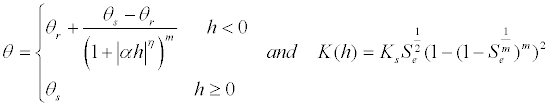(14)

In Equation 14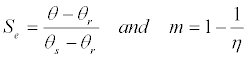(15)

In Equations 14 and 15, θ =soil water content (-), θs=the saturation water content (-), θr=residual water content (-), α (1/L), η (-), and m (-)=soil water characteristics function parameters, K(h)=unsaturated hydraulic conductivity function (L/T), h=pressure head (L), Ks=saturated hydraulic conductivity (L/T), and Se=effective saturation (-).

To conduct the HYDRUS-2D simulation, a computational domain of 1.5 m (horizontal)×3m (vertical) with homogeneous soil profile and initial soil moisture content (Se(qi)=0.184, where qi=initial soil water content) was defined. Because the wetting front did not reach the boundaries of the computational domain during the simulation period (136.0 min), the soil water contents at the boundaries were kept constant during the computation. The computational domain was discretized into 1299 nodes with significantly greater detail around the furrow surface. In addition, the bottom boundary condition was set to free drainage. The depth hydrograph generated by the surface hydraulic model at the furrow inlet was used as the surface boundary condition.

Figure 2 shows a comparison of the cumulative infiltration and infiltration rates computed with HYDRUS-2D and with the numerical approach described here Equations 9 and 10. During the first 25 min of infiltration, cumulative intakes calculated with HYDRUS-2D are larger than those from Equation 9. Nonetheless, the differences are small when compared to the final cumulative infiltration. In the interval subsequent to the first 25 min, cumulative intakes calculated with the two approaches show good agreement until the beginning of recession, after which they diverge. Because intake rates are generally small during the recession phase, these discrepancies did not lead to a significant difference in the computed cumulative intakes. The difference in the final cumulative infiltration computed with the two methods is 0.0018 m3/m, which is only about 2.1% of the cumulative intake obtained with HYDRUS-2D. Note that the noise in intake rates computed with the empirical approach occurs at irrigation phase transitions, for example the largest spike/trough is right after cutoff time. Since they occur over very short time steps, they have little effect on cumulative infiltration. Overall, the preceding discussion suggests that the empirical formulation of furrow infiltration, presented above, is satisfactory.

Figure 2: Comparison of cumulative infiltration and infiltration rate calculated with HYDRUS-2D and Equations 9 and 10.

Evaluation of the numerical solution of the advection equation

Noting the significance of the numerical problems (nonphysical diffusion and oscillations) associated with the discretization of the advection equation, the numerical solution of the advection step is evaluated separately using test problems with steep concentration gradients. The outputs of the numerical model are compared with a simplified analytical model. Assuming flow is steady and uniform, the trajectories (the characteristics) in the (x,t) plane along which concentration is invariant become straight lines. Hence, given solute concentration at any given point in the computational domain, C(x1,t1), the line along which the concentration, C, propagates, unchanged, in the (x,t) plane can be expressed as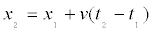(16)

Where (x1,t1) and (x2,t2)=distance and time coordinates (referenced from the furrow inlet and the beginning of irrigation, respectively) of points that are on a characteristic along which the concentration is constant and v=average cross-sectional velocity (L/T) - invariant with time and distance.

In general, flow in an irrigation furrow is unsteady and nonuniform. However, if irrigation is applied to a long, impervious, freedraining furrow for a sufficiently long time, a flow condition that closely approximates a steady uniform flow over a large fraction of the furrow length (excluding a section close to the free over-fall) can be attained. Hence, a hypothetical test case consisting of a furrow with a freedraining downstream end boundary, an impervious bed, and a length of 250 m is set up for this evaluation. The specifics of the hydraulic and transport data used in this evaluation are summarized in Table 1, data set 2. In order to approximate the flow condition for which Equation 16 is valid over the upper 200 m long section of the furrow (80.0% of the test furrow), the hypothetical test furrow was irrigated with pure water (C=0.0 g/m3) at a constant inflow rate of 1.5L/s for 100.0min before solute application begins. Two solute application configurations are considered in the current evaluation Figure 3a and 3c: (1) A step input Figure 3a: with this configuration, the solute concentration at the inlet is set at 0.0 g/m3 for the first 100.0 min following the start of irrigation and then inlet solute concentration is instantly raised to 100.0 g/m3 at t=100.0 min and is maintained constant until inflow cutoff - 200.0 min and (2) A finite pulse input Figure 3c: with this solute input configuration, the furrow is irrigated with pure water for the first 100.0 min. Inlet solute concentration is then raised to 100.0 g/m3 at t=100.0 min and is maintained constant for 15.0 min, then dropping promptly to zero at t=115.0 min. These solute input configurations have nearly infinite spatial concentration gradients at the transitions; hence present the highest degree of difficulty to the numerical solution of the advection equations. Because of this attribute they are commonly used in test problems in evaluating the soundness of the numerical solutions of the advection equation .

Figure 3: Comparison of analytical and numerical solution for pure advection (Kx =0.0m2/sec and Δt=30.0sec) : (a) Step input of 100g/m3 (tS0=100.0min), (b) Solute distribution along the furrow stream at three times -step input, (c) A finite pulse of 100.0g/m3 for 15minutes (ts0=100min and tSco=115.0min), and (d) Solute distribution along the furrow stream at three times – finite pulse input.

Figure 3a and 3c show the solute breakthrough curves at the furrow inlet representing step and finite pulse inputs, respectively. Figure 3b and 3d depict 3D views of the resulting spatial distribution of solute along the furrow, at three different times (116.0 min, 126.0 min, and 136.0 min), calculated with Equation 16 and the numerical model. For both the step input and finite pulse test cases, the amplitude and variance of the solute distribution profiles calculated with the numerical and analytical solutions are in good agreement. These results indicate that the numerical solution of the advection term with a semi- Lagrangian integration scheme is stable and accurate when used in furrow irrigation context.

Comparison of the numerical solution of the advectiondispersion equation with analytical solution

For a steady uniform flow condition, the cross-section averaged advection-dispersion equation can be written as: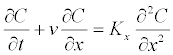(17)

In Equation 17, v and Kx are constants. Given the initial condition (C(x,0)= Ci, for 0 ≤ x ≤ L) and a step solute input at the furrow inlet (C(0,t)=C0, for ts0 ≤ t), where t=current time referenced from the beginning of irrigation (T), and ts0=the time solute application begins, referenced from the beginning of irrigation (T). Equation 17 can be solved analytically :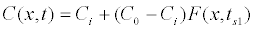(18)

Where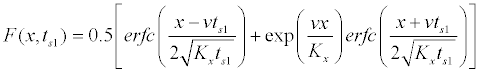(19)

x=distance from inlet end (L) and ts1=t-ts0. Considering a step input at the furrow inlet and a furrow segment closely satisfying the flow conditions for which Equation 17 is valid, the solute breakthrough curve at any given point along the furrow stream or the solute distribution profile with distance can be calculated with Equation 18. For a finite pulse input with a constant concentration, C0, applied over the interval ts0<t<tsco and a zero initial concentration (Ci=0.0g/m3), the solution for Equation 17 in the interval tsco<t is :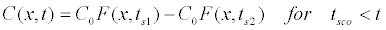(20)

In Equation 20, ts2=ts1 - (tsco- ts0) and tsco=the time at which solute application ends, measured from the beginning of irrigation (T). Note that for ts0<t<tsco, C(x,t) is calculated with Equation 18. Figure 4a shows the finite pulse solute input (i.e., the breakthrough curve at the furrow inlet) used in the current evaluation. Figure 4b depicts a comparison of the spatial solute distribution profiles, predicted by the analytical and numerical models, at four time levels subsequent to the start of solute application (108.0 min, 116.0 min, 121.0 min, and 126.0 min). Noting that actual flow conditions in the test furrow only approximates steady-uniform flow conditions and considering the errors inherent in the numerical solution, the close match obtained between the numerical and analytical solutions Figure 4b validates the accuracy of the numerical model.

Figure 4: Comparison of analytical and numerical solution for 1D advection-dispersion with Kx=0.33 m2/sec, Δt=30.0sec, and tS0=100.0min and tSco=115.0min (a) A finite pulse input of 100.0g/m3 and (b) Solute distribution profiles along the furrow stream.

#### Model Evaluation with Field Data

Description of system parameters

The coupled flow and solute transport model described here requires the specification of field-scale average hydraulic and transport parameters as input data. The hydraulic parameter set includes: the Manning roughness coefficient, n Equation 2, and parameters of the modified Kostiakov infiltration function k, a, and b; Equation 6. The only solute transport parameter of interest here is the hydrodynamic (longitudinal) dispersion coefficient, Kx Equation 11, or constants related to the equation used to estimate Kx. The approaches used to estimate these parameters are briefly described subsequently.

Field experiment

Hydraulic data: In the spring of 2010, a field study was conducted to collect hydraulic and solute transport data in the Desert Research and Extension Center (DREC) of the University of California-Davis. These data sets were collected in sloping free-draining furrows and labeled as DREC-1 and DREC-2 Table 1. The furrows are used to irrigate Alfalfa crop grown on a silty clay loam soil. They are 346.0 m long and have an average longitudinal slope of 0.2%. Irrigation water is delivered to the individual furrows through a gated pipe that runs across the head end of the field. Inflow into individual test furrows was measured with portable flumes. Table 1 presents a summary of the data, including: average inflow rate, inflow cutoff time, furrow length, furrow-wide average geometry parameters and longitudinal slopes. Actual furrow cross-sectional dimensions and longitudinal slopes are spatially variable; however, furrow geometry parameters and slopes shown in Table 1 are spatial averages. In addition, depth hydrographs were measured at six (DREC-1) or five (DREC-2) regularly spaced (≈61m interval) measurement stations along the test furrows. Although the furrow length for the DREC-2 data set as well is 346.0 m, water did not advance beyond 260.0 m during the duration of the experiment (330.0 min) and flow depth measurements were made only over the upper 244.0 m reach of the furrow (the distance at which the fifth depth measurement station was installed). Hence, in subsequent analysis only the upper 244.0 m segment of the furrow is considered and the downstream boundary condition used in the hydraulic simulation is a rating curve defined in terms of the Manning equation, Equation 2.

Solute transport data: In this study the Bromide ion (Br-) is used as a tracer to simulate the transport of soluble nitrogen fertilizer (such as NO3-) in the furrow irrigation stream. A solution of potassium bromide (KBr) in a standard fertilizer feed-tank was placed on an elevated platform close to the head end of the test furrows. The feed tank was connected with a plastic hose to a simple metering apparatus, which injects the Br- solution into the inlet end of a test furrow. The metering apparatus consists of a small box like container fitted with a nozzle at the bottom and a float valve arrangement at the inlet so as to maintain a constant head and hence a constant solution injection rate into the stream. Bromide solution was injected at a rate of about 600 ml/min in five pulses with on-off times of about 30min. Water samples were collected at each of the measurement stations manually at regular time intervals of 10min. The concentration of Br- in the water samples were then measured in the laboratory calorimetrically  with an autoanalyzer . Measured nodal solute breakthrough curves are used to evaluate the solute transport model.

Estimation of soil intake and hydraulic (Infiltration and Manning Roughness) Parameters

Typically, the calibration of surface irrigation hydraulic models is often performed with volume balance based approaches [9,29-31]. These approaches do not explicitly take into account the effects of wetted perimeter variation on furrow in filtration; hence they cannot be applied to the specific formulation of the furrow infiltration problem described here. In this study, furrow infiltration and roughness parameters were estimated with a simple procedure that matches model predicted depth hydrographs with their measured counterparts. Table 1 summarizes the infiltration and roughness parameter estimates for DREC-1 and DREC-2 data sets.

A comparison of measured and model predicted depth hydrographs (computed based on estimates of the infiltration and roughness parameters given in Table 1) for DREC-1 and DREC-2 data set is shown in Figures 5 and 6, respectively. The field-scale Weighted Mean Relative Residual  between the measured and computed flow depth hydrographs are 22.0% and 29.0% for DREC-1 and DREC- 2 data sets, respectively. However, it can be noted that a significant fraction of the WMRR for DREC-2 data set is contributed by the differences between measured and computed depth hydrographs at the downstream computational boundary Figure 6e. In fact, the WMRR (for DREC-2 data set) reduces to 16.0% only if the flow depth data from the downstream computational boundary is excluded from the analysis. This shows that much of the error is associated with the form of the downstream boundary condition. It also shows that the effect of the boundary condition does not extend to a large section of the flow upstream. Note that this observation can be confirmed by a careful look at the hydraulics of furrow irrigation in close vicinity of the downstream boundary, which suggests that the effect of the downstream boundary condition typically attenuates at a short distance upstream.

The use of field measured depth-flow rate data as the downstream boundary condition (instead of the normal depth assumption used in the current analysis) may lead to a better agreement between measured and simulated depth hydrographs for the downstream computational boundary. However, measured rating curve is unavailable for the downstream computational boundary of the DREC-2 data set, hence cannot be used. In addition, note that in a number of instances in Figures 5 and 6, measured nodal peak depths are larger than peak depths measured at an upstream node. These observations are for the most part due to irregularities in the furrow bottom elevation.

Figure 5: Comparison of simulated and measured flow depth hydrographs (DREC-1) at (a) 3.0m, (b) 122.0m, (c) 183.0m, (d) 244.0m, and (d) 305.0m from furrow inlet.

Figure 6: Comparison of simulated and measured flow depth hydrographs (DREC-2) at (a) 3.0m, (b) 61.0m, (c) 122.0m, (d) 183.0m, and (e) 244.0m from furrow inlet.

In general, the parameter estimates (Table 1, DREC-1 and DREC- 2 data sets) imply a high transient intake rate and a low steady state intake rate, which is consistent with the infiltration characteristics of the cracking heavy soil of the study site. In light of the approximate nature of the parameter estimation procedure used and given the uncertainties arising from spatial variability in soil, micro-topography, and furrow geometry not taken into account by the model; these results are deemed acceptable. In theory, more accurate parameter estimates may be obtained if spatial variability is taken into account and a parameter estimation model based on a formal nonlinear optimization algorithm is used.

Solute transport model evaluation

Estimation of longitudinal dispersion coefficient: Accurate estimation of the dispersion coefficient, Kx, is generally difficult . In the current study, Kx is calculated with an approximate equation proposed in reference :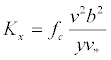(21)

Where fc=a constant (-), v=cross-section average velocity (m/s), b=width of flow (L), y=flow depth (L), and v*=shear velocity (L/T), estimated by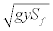, g=gravitational acceleration (L/T2), and Sf=friction slope (-). fc is a constant that depends on the distribution of velocity and depth in a cross-section [23,34]. fc=0.011 was recommended for rivers and canals , however, it has also been shown that fc can vary over a wide range [23,32]. Acceptable match between simulated and measured solute breakthrough curves were obtained for fc=0.011 under furrow irrigated conditions . In this study an initial value of fc=0.011 is used and the sensitivity of the solution to changes in fc was evaluated as well.

Comparison of numerical solution and field data: Figures 7 and 8 compare measured and computed Br- breakthrough curves at six locations (3.0 m, 61.0 mm, 122.0 m, 183.0 m, and 244.0 m and 305.0 m) for DREC-1 and at five locations (3.0 m, 61.0 mm, 122.0 m, 183.0 m, and 244.0m) for DREC-2 data sets. Note that five measured data points, from both DREC-1 and DREC-2 data sets, were deemed outliers, hence excluded from the analysis presented subsequently. In addition, although application of the KBr solution began an hour after the start of irrigation, a small amount of Br- was measured in the water samples collected prior to the start of KBr application (Figures 7 and 8). This could be due to either one or a combination of the following factors: the background concentration of Br- in the irrigation water, noise, Brextant in the soil surface at the time of irrigation gets dissolved by the irrigation water.

Figure 7: Comparison of measured and simulated Br- breakthrough curves (DREC-1) at: (a) 3.0m, (b) 61.0m, (c) 122.0m, (d) 183.0m (e) 244.0m, and (f) 305.0m from furrow inlet.

Figure 8: Comparison of measured and simulated Br- breakthrough curves (DREC-2) at: (a) 3.0m, (b) 61.0m, (c) 122.0m, (d) 183.0m, and (e) 244.0m form furrow inlet.

Figure 7a-7f show that for DREC-1 data set, an fc value of 0.011 resulted in computed breakthrough curves (BTC’s) that are close to pure advection and fc=0.75 led to a more diffused BTC’s over the lower reach of the furrow. The BTC’s corresponding to fc=0.25 are mildly diffused relative to those for fc=0.75. Among the three alternative sets of BTC’s the one corresponding to fc=0.75 provides a marginally better fit to measured data close to the downstream end of the furrow where diffusion appears to be not insignificant. Similarly, for DREC-2 data set Figure 8a-8f, the computed BTC’s corresponding to fc=0.011 are close to pure advection. Slight diffusion can be noted toward the downstream end as fc is increased from 0.011 to 0.25 and then to 0.45. Over the entire length of the furrow, an fc value of 0.011 appears to produce a set of BTC’s that fit measured data better. The maximum relative difference between measured and computed nodal average Brconcentrations (obtained with fc=0.75 for DREC-1 and fc=0.011 for the DREC-2 data sets) is 17.8%, for the DREC-1 data set, and 9.9%, for DREC-2 data set. The furrow-wide Mean Relative Differences (MRD) between the measured and simulated nodal average Br- concentrations, obtained with fc=0.75 for DREC-1 and fc=0.011 for the DREC-2 data sets, are 8.8% and 5.9%, respectively.

In addition, the inlet Br- BTC’s, especially that of the DREC-1 data set, consist of steep concentration gradients and highly irregular patterns, characteristic of a solute transport problem that presents a high degree of difficulty for numerical solutions. Considering this and the uncertainties associated with the factors described above, the fact that the model predicted accurately the location of the BTC’s, the general shape and patterns of the BTC’s as they propagate through the furrow stream, the average nodal solute concentrations, and the furrowwide average solute concentrations with satisfactory accuracy even for a relatively difficult solute transport problem, shows that the model is a valuable predictive tool for fertigation management purposes.

In general, the results presented in Figures 7 and 8, support the emerging consensus that given the relatively short lengths of surface irrigation streams, advection is the dominant solute transport mechanism in surface irrigation. However, it also shows that, if solute is applied in short pulses as opposed to a solute injection pattern in which a constant concentration of solute is applied during the entire irrigation application, hydrodynamic dispersion may not be entirely negligible over the lower reaches of the field Figures 7 and 8. A similar observation was noted in reference  as well.

The simulated longitudinal distribution of water and solute in the soil profile for DREC-1 and DREC-2 data sets is depicted in Figure 9a and 9b. As expected, the longitudinal distribution of water is a monotonic decreasing function of distance. However, the corresponding Br- profile tends to increase over the upper reach of the furrows, peaking at a distance of about 205.0 m and 100.0 m from the furrow inlet for the DREC-1 and DREC-2 data sets, respectively, and then decreasing over the lower section of the furrows. The observed trend, in the Br- profile, over the upper segment of the furrows is mainly due to the differences in the water and solute advance times to the computational nodes there, which is maximum at the upstream end and decreases rapidly with distance from inlet end. For soils with a very high transient intake rates compared to the steady state intake rates (like the DREC-1 and DREC-2 data sets) the infiltration that occurs in a relatively short period immediately following initial wetting of a computational node represents a major fraction of the nodal cumulative infiltration, hence differences between water and solute advance times have a significant effect on nodal cumulative intake of solutes. On the hand, in the lower section of the furrow the amount of solute in the subsurface decreases with distance mainly due to a combination of smaller intake opportunity times and reduced solute concentrations in the irrigation stream (Figures 7e, 7f, 8d, and 8e).

Figure 9: Simulated longitudinal infiltration profile of irrigation applied water and bromide (as a function of longitudinal dispersion constant, fc): (a) DREC-1 and (b) DREC-2.

Figures 9a and 9b also show the effects of dispersion on the longitudinal subsurface profile of solutes as fc is increased from 0.011 to 0.75 for DREC-1 and from 0.011 to 0.45 for DREC-2 data sets. The effect of hydrodynamic dispersion is insignificant in the upper reaches of the test furrows and becomes relatively more pronounced toward the downstream end of the furrow. This is consistent with the fact that significant solute dispersion occurs only with distance. In addition, the results suggest that the longitudinal distribution uniformity of infiltrated bromide tends to improve as the dispersion coefficient is increased. However, the change in the subsurface longitudinal distribution of solutes is less significant than would be expected considering the amount of increase in the fc values.

Note that for the given geometric and hydraulic parameter sets, the dispersion coefficient appears to be less sensitive to changes in fc, which may explain the relatively small spread in the solute BTC’s associated with fc values of 0.45 and 0.75 (Figures 7 and 8) and the corresponding subsurface Br- distribution profiles (Figure 9). In addition, it can be noted that the effect of hydrodynamic dispersion on solute infiltration and hence the subsurface longitudinal distribution profile of solutes could be related to soil intake characteristics. The simulation results for DREC-1 and DREC-2 data sets suggests that in heavy soils hydrodynamic dispersion has a more significant effect on the Br amount in surface runoff than on the longitudinal subsurface Br distribution profile. For instance, for DREC-1 data set Br in tail water runoff increased from 73.3 g for fc=0.011 to 86.6 g for fc=0.75. For DREC-2 data set, however, Br- in runoff showed only a small increase from 29.8 g (fc=0.011) to 31.1 g (fc=0.45). This could be mainly due to the fact that in the DREC-2 furrow only a much smaller fraction of the applied Br reached the downstream computational boundary compared to that of DREC-1 furrow (Figures 7f and 8e).

#### Summary and Conclusions

A coupled flow and solute transport model for irrigation furrows is evaluated with field data. A simple procedure that matches computed and measured flow depth hydrographs was used to estimate infiltration and roughness parameters. An explicit equation is used to approximate longitudinal dispersion coefficient as a function of geometry and hydraulic parameters. Cumulative infiltration and rate of infiltration computed with the numerical approach proposed here are in good agreement with those predicted by HYDRUS-2D. Solute distribution profiles computed with the numerical solute transport model under special flow (quasi-uniform) conditions compare well with those obtained using simplified analytical solutions for pure advection and 1D advection-dispersion.

The coupled model predicted with satisfactory accuracy the location of the measured breakthrough curves (BTC’s), the general shape and patterns of the BTC’s as they propagate through the furrow stream, the average nodal solute concentrations, and the furrow-wide average solute concentrations. This is significant, because these factors are good indicators of the longitudinal subsurface distribution of solutes and hence the performance of a surface fertigation event. Considering the spatial variability of system properties not explicitly taken into account by the model and the complex configuration of the solute BTC’s, the results suggest that the coupled flow-transport model is overall a valuable predictive tool for irrigation/fertigation system management.

Results also suggest that model prediction could be improved if the hydraulic model is further developed to take into account spatial variability of system properties. In addition, the availability of a furrow irrigation hydraulic parameter estimation model that takes into account furrow wetted perimeter variation in the framework of a formal mathematical programming algorithm could improve accuracy of model predictions.

#### References

Select your language of interest to view the total content in your interested language

### Article Usage

• Total views: 12809
• [From(publication date):
August-2014 - Dec 16, 2019]
• Breakdown by view type
• HTML page views : 8928Can't read the image? click here to refresh